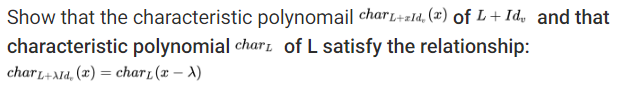Show that the characteristic polynomail ch of L Id and thatcharacteristic polynomial chari of L satisfy the relationship:charl+Ald, (z) = charL (z-A)

Questionhelp_outlineImage TranscriptioncloseShow that the characteristic polynomail ch of L Id and that characteristic polynomial chari of L satisfy the relationship: charl+Ald, (z) = charL (z-A) fullscreen
Step 1

To prove  the relationship  between the  characteristic polynomials of the two given transformations

Step 2

Let c be an eigenvalue of L with an eigenvector v (not 0) by definition. We prove  that c+λ is an eigenvalue of L+λI with eigenvector v

Step 3

The characteristic polynomial of any linear operator is dete...

Want to see the full answer?

See Solution

Want to see this answer and more?

Our solutions are written by experts, many with advanced degrees, and available 24/7

See Solution
Tagged in

Math